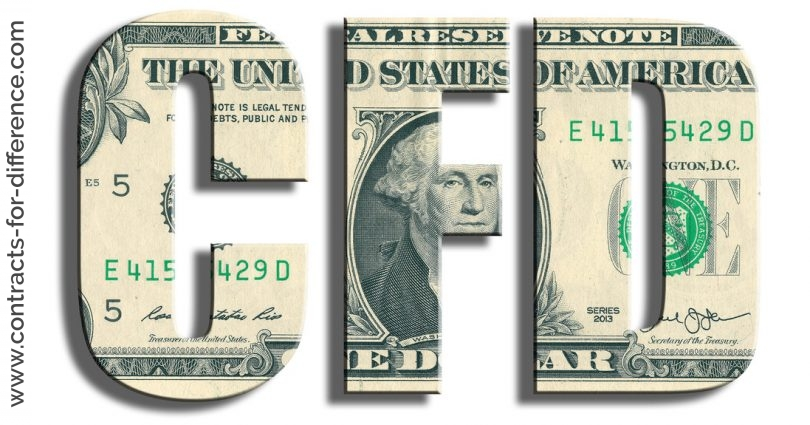> > Value of One CFD

# Value of One CFDWritten by

## What is the Value of one CFD and in what Currency is this Traded in?

A single contract between you and your CFD provider has the same value as buying one share in the underlying company.

The full value of your CFD position is represented by your stake (or quantity of CFDs you buy) * the price:

CFDs are traded in the base currency of the contract as you will see from the examples given below. When trading CFDs you do not need to pay the full value of your market exposure. Let’s see how this works with some examples -:

## One Share CFD

If you buy share CFDs these are valued in the 1/100 of the value of the basic monetary unit.

## One CFD in Forex

If you buy a Forex CFD this has the value of the quote currency and is valued in the quoted currency.

Buying one contract in GBP (base currency)/ USD will have the value of 1 USD * the price. Example: Buy 6 CFDs of GBP/USD at 1.1322 = 6 * 11322 = a full value of \$67,932.

Buying one contract in EU (base currency)/ GBP will have the value of 1 Pound*the price. Example: Buy 6 CFDs of EUR/GBP at 0.9217 = 6 * 9217 = a full value of £55,302.

Buying one contract in USD (base currency)/ EU will have the value of 1 EUR*the price. Example: Buy 6 CFDs of USD/EUR at 0.7455= 6 * 7455= a full value of €44,730.

## One Contract in Indexes

If you buy a single index contract for difference, this has the value of the basic monetary unit and it is valued in the quoted currency.

One contract on the FTSE (UK Index) is equivalent to a market exposure of 1 Pound * The Price. Example: Buy 10 CFDs of FTSE at £4450. The full value of the trade is: 10 * £4450 = £44,500.

One contract on the Dax 30 (EU Index) is equivalent to a market exposure of 1 Euro * The Price. Example: Buy 15 CFDs of DAX at €4312. The full value of the trade is: 15 * €4312= €64,680.

One contract on the S&P 500 (US Index) is equivalent to a market exposure of 1 USD * The Price. Example: Buy 50 CFDs of S&P 500 at \$950.20. The full value of the trade is: 50 * \$950.20 = \$47,510.

One contract on the Nikkei (Japanese Index) is equivalent to a market exposure of 1 JPY * The Price. Example: Buy 150 CFDs of Nikkei at ¥ 7842. The full value of the trade is: 150 * ¥ 7842 = ¥ 1176300.

## One Contract in Commodities

If you buy a commodity CFD this has the value of the basic monetary unit it is quoted in and is valued in the quoted currency. Example: One CFD in the Gold @ 970 has a value of \$970. Buy 2 CFD in the US Oil @ 82.56 has a value of \$16,512.